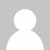I’m still enjoying solving the Advent of Code daily challenges. Here’s a video of how I tackled day 4, with the code below

Solution in C#

``````var secretKey = "iwrupvqb"; //"pqrstuv"; //"abcdef";
var md5 = System.Security.Cryptography.MD5.Create();
var q = from n in Enumerable.Range(1, 10000000)
let inputString = \$"{secretKey}{n}"
let inputBytes = System.Text.Encoding.ASCII.GetBytes(inputString)
let hashBytes = md5.ComputeHash(inputBytes)
let hashString = BitConverter.ToString(hashBytes).Replace("-","")
where hashString.StartsWith("00000") // a: five zeroes, b: six zeroes
select new { n, hashString };
q.FirstOrDefault().Dump();
``````

Solution in F#

``````let secretKey = "iwrupvqb" //"pqrstuv"; //"abcdef";
let prefix = "00000"
let md5 = System.Security.Cryptography.MD5.Create()
let q = seq {
for n in 1 .. 10000000 do
let inputString = sprintf "%s%d" secretKey n
let inputBytes = System.Text.Encoding.ASCII.GetBytes(inputString)
let hashBytes = md5.ComputeHash(inputBytes)
let hashString = BitConverter.ToString(hashBytes).Replace("-","")
if hashString.StartsWith(prefix) then yield (n,hashString)
}
``````
Want to learn more about LINQ? Be sure to check out my Pluralsight course LINQ Best Practices.How did you choose the correct upper bound without knowing the answer first ?
there is Seq.initInfinite in F# (which can be easily written in C# too)
Also MD5 is an IDisposable class it would have been nice to dispose it (using "using" [or use in F#])
Why yielding the hashed string when not needed in the answer ; technically the code is wrong (or need a fst call or anonymous property access before Dump)
I personnaly don't convert the byte array to string ; (ab)using pattern matching to search the pattern required (because it was some "easy" pattern)

``let common matchPattern =    use md5 = Cryptography.MD5.Create ()    Seq.initInfinite ((+) 1 >> sprintf "iwrupvqb%d")    |> Seq.findIndex (         Encoding.ASCII.GetBytes      >> md5.ComputeHash      >> matchPattern)    |> (+) 1 // index starts at 0 when answer starts at 1let part1 () = common (Array.take 3 >> function [|0uy; 0uy; b|] -> b &&& 0xF0uy = 0uy | _ -> false)let part2 () = common (Array.take 3 >> function [|0uy; 0uy; 0uy|] -> true | _ -> false)``

Sehnsuchtyes, the upper bound was arbitrary - just to stop me looping forever if I made a mistake. And yes, converting to string before matching the start is a bit lazy I guess!
thanks again for the tips, it really helps

Mark Heath